# Find First Cell with Any Value – Excel & Google Sheets

This tutorial will demonstrate how to find the first non-blank cell in a range in Excel and Google Sheets.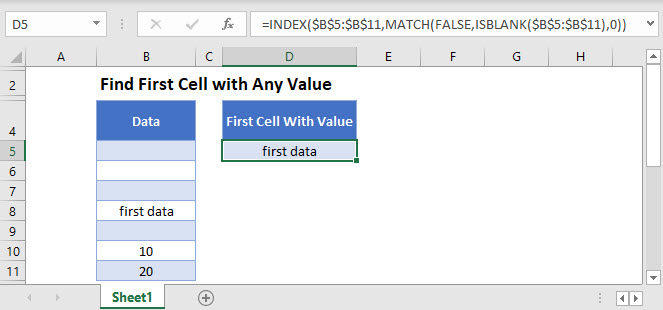## Find First Non-Blank Cell

You can find the first non-blank cell in a range with the help of the ISBLANK, MATCH, and INDEX Functions.

``=INDEX(B3:B10,MATCH(FALSE,ISBLANK(B3:B10),0))``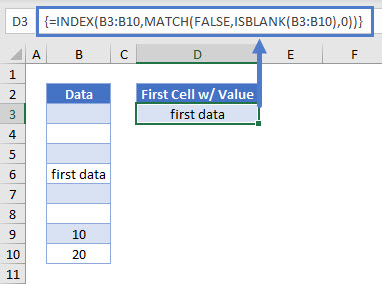Note: This is an array formula. If you are using Excel 2019 or earlier, you must enter the formula with CTRL + SHIFT + ENTER instead of just ENTER.

Let’s see how this formula is built up.

### ISBLANK Function

The ISBLANK Function analyzes every cell in the B3:B11 range and returns an array of TRUE and FALSE values. In our example it would look like this:

``{FALSE, FALSE, FALSE, TRUE, FALSE, FALSE, TRUE, TRUE}``

### MATCH Function

With the MATCH Function we determine the position of the first TRUE value in the array created by the ISBLANK Function.

``=MATCH(FALSE,ISBLANK(B3:B10),0)``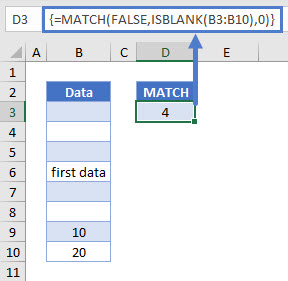### INDEX Function

Using the result of the MATCH Function we can determine with the INDEX Function the row of the first non-blank cell in the range and return its value.

``=INDEX(B3:B10,D3)``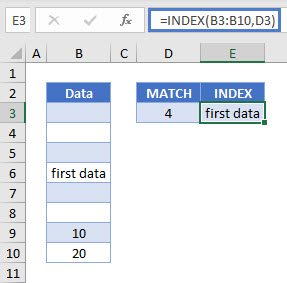Substituting the Cell D3 reference with our MATCH formula give us our original formula:

``=INDEX(B3:B10,MATCH(FALSE,ISBLANK(B3:B10),0))``

Reminder: This is an array formula. If you are using Excel 2019 or earlier, you need to press CTRL + SHIFT + ENTER to enter the formula, instead of simply pressing ENTER.

## Find First Cell With Any Value in Google Sheets

This formula works the same in Google Sheets except that it’s not necessary to hit CTRL + SHIFT + ENTER.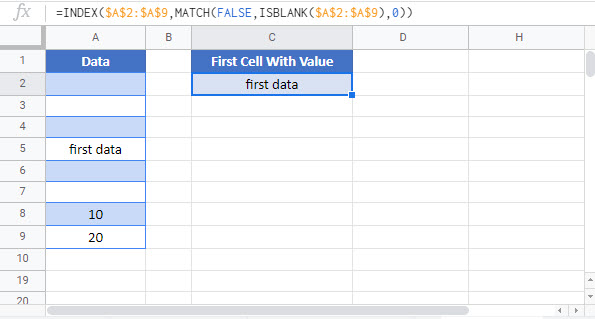### Excel Practice Worksheet

Practice Excel functions and formulas with our 100% free practice worksheets!